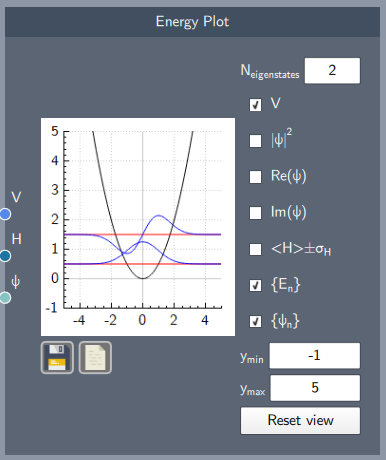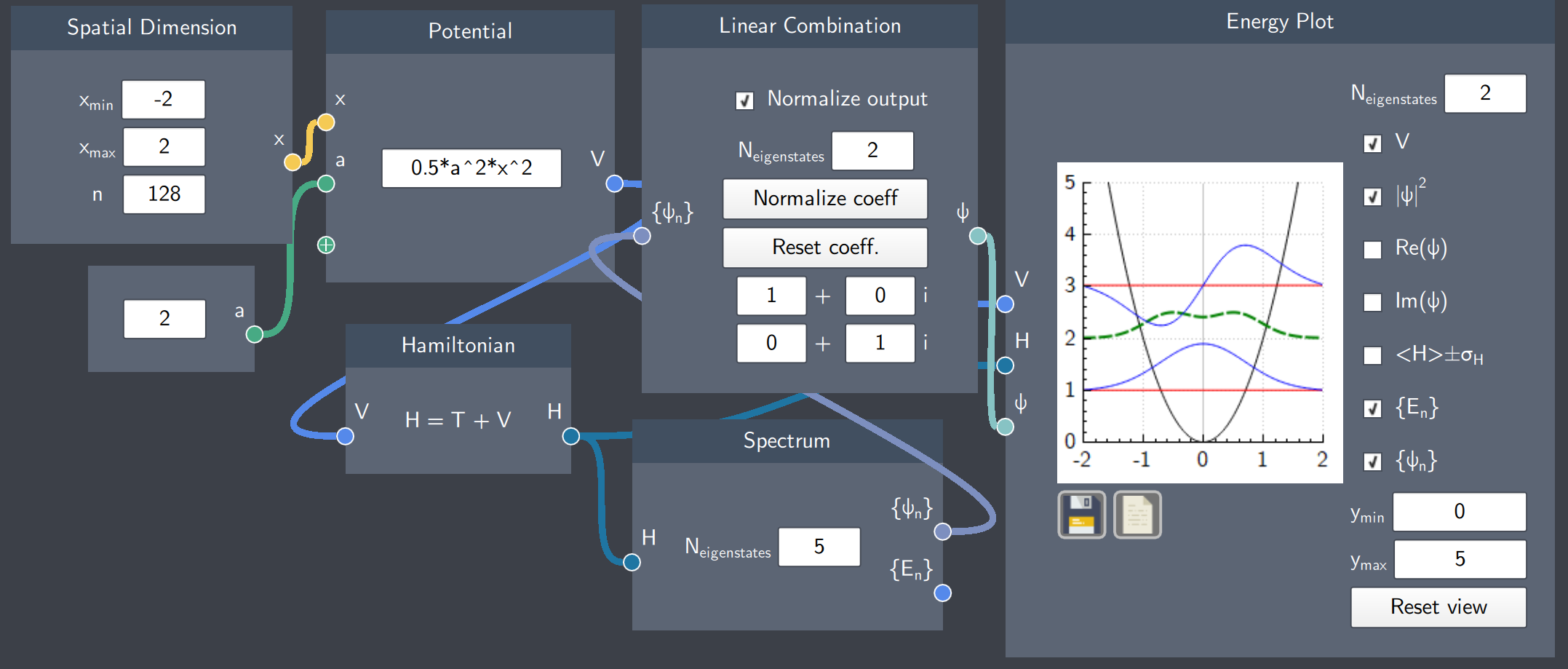# Energy Plot

## Description

The Energy Plot node plots the eigenstates and associated eigenvalues, the potential function, probability density, real and imaginary parts of a wave function, as well as the expectation value of the Hamiltonian (\langle H \rangle) and its standard deviation.## Input

The node has the following inputs:

• Potential ($V$): The Potential node where the potential function is defined
• Hamiltonian (H): The Hamiltonian node
• Wave Function ($\psi$): A wave function

## Content

This plot consists of check-boxes corresponding to different functions, which can be selected to visualize them. In the $N_{eigenstates}$ content-field, the desired number of eigenstates and eigenvalues is entered.

The different check-boxes/functions from top to bottom (with required inputs in parenthesis) are:

• Show/Hide the potential (V)
• Show/Hide the probability distribution of the state ($\psi$)
• Show/Hide the real part of the state ($\psi$)
• Show/Hide the imaginary part of the state ($\psi$)
• Show/Hide the expecation value of the Hamiltonian +/- the standard deviation (H)
• Show/Hide the eigenvalues (H)
• Show/Hide the eigenstates (H)

Additionally, the $y$-axis limits can be set to any limits and reset to the original scale.  The plot can be saved and the data can be exported as a $.csv$ file.

## Output

The output of the node is the visualization in the plot based on the inputs and selection of the check-boxes.

## Example

In the example below, the harmonic oscillator potential has been plotted along with a superposition state (linear combination of the first two eigenstates of the harmonic oscillator), eigenstates and eigenvalues as selected in the check-boxes. The probability density is denoted by a green dashed-curve. As the $N_{eigenstates}$ = 2, the first two eigenstates (blue curves) and eigenvalues (red lines) are plotted.